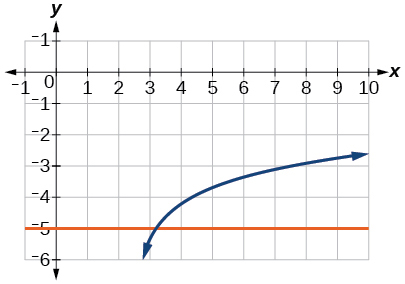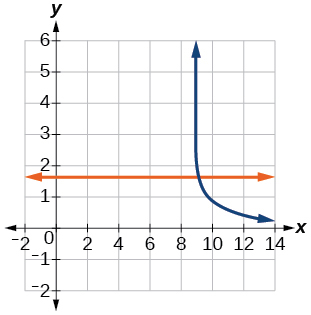# 4.6E: Exercises

$$\newcommand{\vecs}{\overset { \rightharpoonup} {\mathbf{#1}} }$$ $$\newcommand{\vecd}{\overset{-\!-\!\rightharpoonup}{\vphantom{a}\smash {#1}}}$$$$\newcommand{\id}{\mathrm{id}}$$ $$\newcommand{\Span}{\mathrm{span}}$$ $$\newcommand{\kernel}{\mathrm{null}\,}$$ $$\newcommand{\range}{\mathrm{range}\,}$$ $$\newcommand{\RealPart}{\mathrm{Re}}$$ $$\newcommand{\ImaginaryPart}{\mathrm{Im}}$$ $$\newcommand{\Argument}{\mathrm{Arg}}$$ $$\newcommand{\norm}{\| #1 \|}$$ $$\newcommand{\inner}{\langle #1, #2 \rangle}$$ $$\newcommand{\Span}{\mathrm{span}}$$ $$\newcommand{\id}{\mathrm{id}}$$ $$\newcommand{\Span}{\mathrm{span}}$$ $$\newcommand{\kernel}{\mathrm{null}\,}$$ $$\newcommand{\range}{\mathrm{range}\,}$$ $$\newcommand{\RealPart}{\mathrm{Re}}$$ $$\newcommand{\ImaginaryPart}{\mathrm{Im}}$$ $$\newcommand{\Argument}{\mathrm{Arg}}$$ $$\newcommand{\norm}{\| #1 \|}$$ $$\newcommand{\inner}{\langle #1, #2 \rangle}$$ $$\newcommand{\Span}{\mathrm{span}}$$$$\newcommand{\AA}{\unicode[.8,0]{x212B}}$$

## 4.6: Exponential and Logarithmic Equations

Uncontrolled population growth can be modeled with exponential functions. Equations resulting from those exponential functions can be solved to analyze and make predictions about exponential growth. In this section, we will learn techniques for solving exponential functions.

### Verbal

1) How can an exponential equation be solved?

Determine first if the equation can be rewritten so that each side uses the same base. If so, the exponents can be set equal to each other. If the equation cannot be rewritten so that each side uses the same base, then apply the logarithm to each side and use properties of logarithms to solve.

2) When does an extraneous solution occur? How can an extraneous solution be recognized?

3) When can the one-to-one property of logarithms be used to solve an equation? When can it not be used?

The one-to-one property can be used if both sides of the equation can be rewritten as a single logarithm with the same base. If so, the arguments can be set equal to each other, and the resulting equation can be solved algebraically. The one-to-one property cannot be used when each side of the equation cannot be rewritten as a single logarithm with the same base.

### Algebraic

For the following exercises, use like bases to solve the exponential equation.

4) $$4^{-3v-2}=4^{-v}$$

5) $$64\cdot 4^{3x}=16$$

$$x=-\dfrac{1}{3}$$

6) $$3^{2x+1}\cdot 3^x=243$$

7) $$2^{-3n}\cdot \dfrac{1}{4}=2^{n+2}$$

$$n=-1$$

8) $$625\cdot 5^{3x+3}=125$$

9) $$\frac{36^{3b}}{36^{2b}}=216^{2-b}$$

$$b=\dfrac{6}{5}$$

10) $$\left (\dfrac{1}{64} \right )^{3n}\cdot 8=2^6$$

For the following exercises, use logarithms to solve.

11) $$9^{x-10}=1$$

$$x=10$$

12) $$2e^{6x}=13$$

13) $$e^{r+10}-10=-42$$

No solution

14) $$2\cdot 10^{9a}=29$$

15) $$-8\cdot 10^{p+7}-7=-24$$

$$p=\log \left (\frac{17}{8} \right )-7$$

16) $$7e^{3n-5}+5=-89$$

17) $$e^{-3k}+6=44$$

$$k=-\frac{\ln(38)}{3}$$

18) $$-5e^{9x-8}-8=-62$$

19) $$-6e^{9x+8}+2=-74$$

$$x=\frac{\frac{\ln(38)}{3}-8}{9}$$

20) $$2^{x+1}=5^{2x-1}$$

21) $$e^{2x}-e^{x}-132=0$$

$$x=\ln 12$$

22) $$7e^{8x+8}-5=-95$$

23) $$10e^{8x+3}+2=8$$

$$x=\frac{\frac{\ln(3)}{5}-3}{8}$$

24) $$4e^{3x+3}-7=53$$

25) $$8e^{-5x-2}-4=-90$$

No solution

26) $$3^{2x+1}=7^{x-2}$$

27) $$e^{2x}-e^{x}-6=0$$

$$x=\ln 3$$

28) $$3e^{3-3x}+6=-31$$

For the following exercises, use the definition of a logarithm to rewrite the equation as an exponential equation.

29) $$\log \left ( \frac{1}{100} \right )=-2$$

$$10^{-2}=\dfrac{1}{100}$$

30) $$\log _{324}(18)=\dfrac{1}{2}$$

For the following exercises, use the definition of a logarithm to solve the equation.

31) $$5\log _{7}n=10$$

$$n=49$$

32) $$-8\log _{9}x=16$$

33) $$4+\log _{2}(9k)=2$$

$$k=\dfrac{1}{36}$$

34) $$2\log (8n+4)+6=10$$

35) $$10-4\ln (9-8x)=6$$

$$x=\dfrac{9-e}{8}$$

For the following exercises, use the one-to-one property of logarithms to solve.

36) $$\ln (10-3x)=\ln (-4x)$$

37) $$\log_{13} (5n-2)=\log_{13} (8-5n)$$

$$n=1$$

38) $$\log (x+3)-\log (x)=\log (74)$$

39) $$\ln (-3x)=\ln (x^2-6x)$$

No solution

40) $$\log_4 (6-m)=\log_4 (3m)$$

41) $$\ln (x-2)-\ln (x)=\ln (54)$$

No solution

42) $$\log_9 (2n^2-14n)=\log_9 (-45+n^2)$$

43) $$\ln (x^2-10)+\ln (9)=\ln (10)$$

$$x=\pm \frac{10}{3}$$

For the following exercises, solve each equation for $$x$$.

44) $$\log (x+12)=\log (x)+\log (12)$$

45) $$\ln (x)+\ln (x-3)=\ln (7x)$$

$$x=10$$

46) $$\log_2 (7x+6)=3$$

47) $$\ln (7)+\ln (2-4x^2)=\ln (14)$$

$$x=0$$

48) $$\log_8 (x+6)-\log_8 (x)=\log_8 (58)$$

49) $$\ln (3)-\ln (3-3x)=\ln (4)$$

$$x=\dfrac{3}{4}$$

50) $$\log_3 (3x)-\log_3 (6)=\log_3 (77)$$

### Graphical

For the following exercises, solve the equation for $$x$$$,$if there is a solution. Then graph both sides of the equation, and observe the point of intersection (if it exists) to verify the solution.

51) $$\log_9 (x)-5=-4$$

$$x=9$$52) $$\log_3 (x)+3=2$$

53) $$\ln (3x)=2$$

$$x=\dfrac{e^2}{3}\approx 2.5$$54) $$\ln (x-5)=1$$

55) $$\log (4)+\log (-5x)=2$$

$$x=-5$$56) $$-7+\log_3 (4-x)=-6$$

57) $$\ln (4x-10)-6=-5$$

$$x=\dfrac{e+10}{4}\approx 3.2$$58) $$\log (4-2x)=\log (-4x)$$

59) $$\log_{11} (-2x^2 -7x)=\log_{11} (x-2)$$

No solution60) $$\ln (2x+9)=\ln (-5x)$$

61) $$\log_9 (3-x)=\log_9 (4x-8)$$

$$x=\dfrac{11}{5}\approx 2.2$$62) $$\log (x^2+13)=\log (7x+3)$$

63) $$\dfrac{3}{\log _2(10)}-\log (x-9)=\log (44)$$

$$x=\dfrac{101}{11}\approx 9.2$$64) $$\ln (x)-\ln (x+3)=\ln (6)$$

For the following exercises, solve for the indicated value, and graph the situation showing the solution point.

65) An account with an initial deposit of $$\6,500$$ earns $$7.25\%$$ annual interest, compounded continuously. How much will the account be worth after $$20$$ years?

about $$\27,710.24$$66) The formula for measuring sound intensity in decibels $$D$$ is defined by the equation $$D=10\log \left ( \frac{I}{I_0} \right )$$$,$where $$I$$ is the intensity of the sound in watts per square meter and $$I_0=10^{-12}$$ is the lowest level of sound that the average person can hear. How many decibels are emitted from a jet plane with a sound intensity of $$8\cdot 3\cdot 10^2$$ watts per square meter?

67) The population of a small town is modeled by the equation $$P=1650e^{0.5t}$$ where $$t$$ is measured in years. In approximately how many years will the town’s population reach $$20,000$$?

about $$5$$ years### Technology

For the following exercises, solve each equation by rewriting the exponential expression using the indicated logarithm. Then use a calculator to approximate the variable to $$3$$ decimal places.

68) $$1000(1.03)^t=5000$$ using the common log.

69) $$e^{5x}=17$$ using the natural log.

$$\dfrac{\ln (17)}{5}\approx 0.567$$

70) $$3(1.04)^{3t}=8$$ using the common log

71) $$3^{4x-5}=38$$ using the common log

$$x=\dfrac{\log (38+5\log (3))}{4\log(3)}\approx 2.078$$

72) $$50e^{-0.12t}=10$$ using the natural log

For the following exercises, use a calculator to solve the equation. Unless indicated otherwise, round all answers to the nearest ten-thousandth.

73) $$7e^{3x-5}+7.9=47$$

$$x\approx 2.2401$$

74) $$\ln (3)+\ln (4.4x+6.8)=2$$

75) $$\log(-0.7x-9)=1+5\log(5)$$

$$x\approx -44655.7143$$

76) Atmospheric pressure $$P$$ in pounds per square inch is represented by the formula $$P=14.7e^{-0.21x}$$$,$ where $$x$$ is the number of miles above sea level. To the nearest foot, how high is the peak of a mountain with an atmospheric pressure of $$8.369$$ pounds per square inch? (Hint: there are $$5280$$ feet in a mile)

77) The magnitude $$M$$ of an earthquake is represented by the equation $$M=\dfrac{2}{3}\log \left ( \dfrac{E}{E_0} \right )$$ where $$E$$ is the amount of energy released by the earthquake in joules $$E_0=10^{4.4}$$ and is the assigned minimal measure released by an earthquake. To the nearest hundredth, what would the magnitude be of an earthquake releasing $$1.4\cdot 10^{13}$$ joules of energy?

about $$5.83$$

### Extensions

78) Use the definition of a logarithm along with the one-to-one property of logarithms to prove that $$b^{\log_b x}=x$$.

79) Recall the formula for continually compounding interest, $$y=Ae^{kt}$$$.$Use the definition of a logarithm along with properties of logarithms to solve the formula for time $$t$$ such that $$t$$ is equal to a single logarithm.

$$t=\ln \left ( \left ( \dfrac{y}{A} \right )^{\frac{1}{k}} \right )$$

80) Recall the compound interest formula $$A=a\left ( 1+\frac{r}{k} \right )^{kt}$$$.$Use the definition of a logarithm along with properties of logarithms to solve the formula for time $$t$$

81) Newton’s Law of Cooling states that the temperature $$T$$ of an object at any time $$t$$ can be described by the equation $$T=T_s+(T_0-T_s)e^{-kt}$$$,$ where $$T_s$$ is the temperature of the surrounding environment, $$T_0$$ is the initial temperature of the object, and $$k$$ is the cooling rate. Use the definition of a logarithm along with properties of logarithms to solve the formula for time $$t$$ such that $$t$$ is equal to a single logarithm.

$$t=\ln \left ( \left ( \frac{T-T_s}{T_0-T_s} \right )^{-\frac{1}{k}} \right )$$